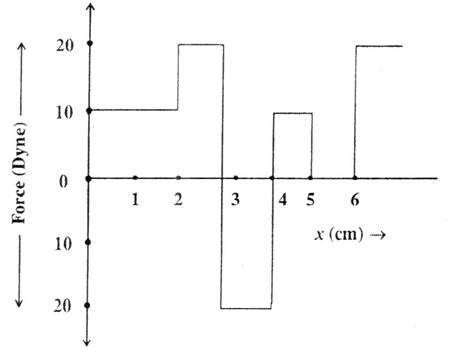# WORK ENERGY AND POWER

• Home -
• WORK ENERGY AND POWER

WORK ENERGY AND POWER - Topic Wise Multiple Choice Questions (MCQ)

Topic Wise free Work Energy and Power MCQ with solutions for 11th 12th exam Practice Multiple Choice Questions and answer for JEE-mains State CET Engineering Entrance GATE free Online Test

1 )

How much is the work done in pulling up a block of wood weighing 2KN for a length of 10m on a smooth plane inclined at an angle of 30° with the horizontal ?

1.732 KJ
17.32 KJ
10 KJ
100 KJ

View Solution

2 )

A force of 7N, making an angle θ with the horizontal, acting on an object displaces it by 0.5m along the horizontal direction. If the object gains K.E. of 2J, what is the horizontal component of the force?

2N
4N
1N
14N

View Solution

3 )

A 60 kg JATAN with 10 kg load on his head climbs 25 steps of 0.20m height each. What is the work done in climbing? (g = 10 m/s2)

3500 J
350 J
35 J
4000 J

View Solution

4 )

A ball of mass 5 kg is striding on a plane with initial velocity of 10 m/s. If co-efficient of friction between surface and ball is (1/2), then before stopping it will describe ……. (g = 10 m/s2)

15 m
17 m
7 m
10 m

View Solution

5 )

The relationship between force and position is shown in the figure given (in one dimensional case) calculate the work done by the force in displacing a body from x = 0 cm to x = 5 cm30 ergs
70 ergs
20 ergs
60 ergs

View Solution